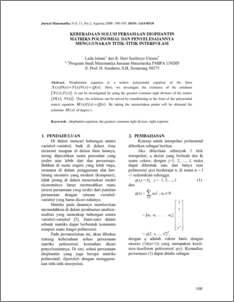# KEBERADAAN SOLUSI PERSAMAAN DIOPHANTIN MATRIKS POLINOMIAL DAN PENYELESAIANNYA MENGGUNAKAN TITIK-TITIK INTERPOLASI

Istiani, Laila and Soelistyo Utomo2, R. Heri (2008) KEBERADAAN SOLUSI PERSAMAAN DIOPHANTIN MATRIKS POLINOMIAL DAN PENYELESAIANNYA MENGGUNAKAN TITIK-TITIK INTERPOLASI. JURNAL MATEMATIKA DAN KOMPUTER, 11 (2). pp. 100-105. ISSN 1410-8518Preview
PDF
123Kb

## Abstract

Diophantine equation is a matrix polynomial equation of the form . Here, we investigate the existence of the solutions . It can be investigated by using the grestest common right divisors of the matrix . Then, the solutions can be solved by transforming to the form of the polynomial matrix equation . By taking the interpolation points will be obtained the solutions of degree r.

Item Type: Article diophantin equation, the greatest common right divisor, right coprime Q Science > QA Mathematics Faculty of Science and Mathematics > Department of Mathematics 1951 INVALID USER 01 Dec 2009 01:20 04 Dec 2009 08:52

Repository Staff Only: item control page